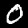# Image Classification Example

Image classification refers to the task of extracting information classes from an image.

In this example, you learn how to implement inference code with Deep Java Library (DJL) to recognize handwritten digits from an image.

The image classification example code can be found at ImageClassification.java.

You can also use the Jupyter notebook tutorial. The Jupyter notebook explains the key concepts in detail.

## Run the image classification example

The model you can use is generated by the training example. Run the training example to generate the model before continuing with this example. The trained model will be stored as `build/model/mlp-XXXX.params`.

### Input image file

In the previous training example, you trained the model using the grayscale handwritten digit dataset, MNIST. You can find the following image in your project test resource folder: `src/test/resources/0.png`### Build the project and run

Run the project by using the following command:

``````cd examples
``````

Your output should look like the following:

``````[INFO ] - [
class: "0", probability: 0.99996
class: "2", probability: 0.00002
class: "6", probability: 5.4e-06
class: "9", probability: 2.5e-06
class: "8", probability: 8.5e-07
]
``````

The results show that there’s a 99.996 percent probability that the image contains a “0” digit.